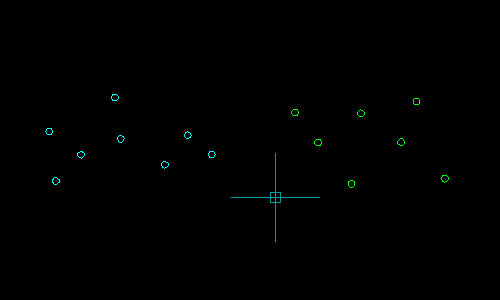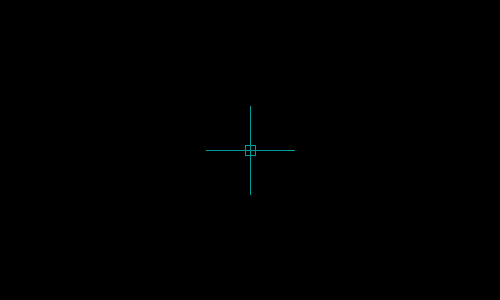# Minimum Enclosing Circle

 Function Syntax (LM:MinEncCircle ) Current Version 1.0 Download MinEncCircle.lsp View HTML Version MinEncCircle.html
Arguments
Symbol Type Description
lst List List of Points to process
Returns
Type Description
List List of  (<centre> <radius>)  of Minimum Enclosing Circle

## Program Description

The Minimum Enclosing Circle problem, (also known as the Smallest Circle problem, or Minimum Covering Circle problem), is the mathematical problem of computing the smallest circle that contains every point in a given set of points in the plane.

The problem was first proposed by the English mathematician James Joseph Sylvester in 1857 and many algorithms have since been devised to compute the solution to the problem.

The Minimum Enclosing Circle (MEC) of a point set is unique and can be determined by at most three points in the set which lie on the circumference of the circle. If the MEC is determined by only two points, then the line joining these points is the diameter of the circle.

The function displayed on this page implements an algorithm by Pr.Chrystal, published in 1885. This algorithm utilises the fact that points defining the MEC will lie on the boundary of the Convex Hull of the point set.

The function hence first computes the Convex Hull of the point set, then repeatedly reduces the radius of the circle until the Minimum Enclosing Circle is reached. The Convex Hull of the point set is calculated using the Graham Scan Algorithm, implemented by my Convex Hull function.

A couple of test programs are displayed below to demonstrate the function.

## Test Function 1

This function will prompt the user to make a selection of AutoCAD Point Entities, then proceed to create the unique Minimum Enclosing Circle enclosing all points in the selection.

### Preview of Test Function 1Select all
```(defun c:test1 ( / i l s )
(if
(and
(setq s (ssget '((0 . "POINT"))))
(setq l
(LM:MinEncCircle
(repeat (setq i (sslength s))
(setq l (cons (cdr (assoc 10 (entget (ssname s (setq i (1- i)))))) l))
)
)
)
)
(entmake (list '(0 . "CIRCLE") (cons 10 (car l)) (cons 40 (cadr l))))
)
(princ)
)
```

## Test Function 2

This function will continuously prompt the user to pick a point whilst displaying the unique Minimum Enclosing Circle around the selected point and all previously selected points.

### Preview of Test Function 2Select all
```(defun c:test2 ( / c e l p )
(if (setq p (getpoint "\nSpecify First Point: "))
(progn
(setq p (trans p 1 0)
l (cons  p l)
)
(entmake (list '(0 . "POINT") (cons 10 p)))
(while (setq p (getpoint "\nSpecify Next Point: "))
(if e
(progn (entdel e) (setq e nil))
)
(setq p (trans p 1 0)
l (cons  p l)
)
(entmake (list '(0 . "POINT") (cons 10 p)))
(if (setq c (LM:MinEncCircle l))
(setq e (entmakex (list '(0 . "CIRCLE") (cons 10 (car c)) (cons 40 (cadr c)))))
)
)
)
)
(princ)
)